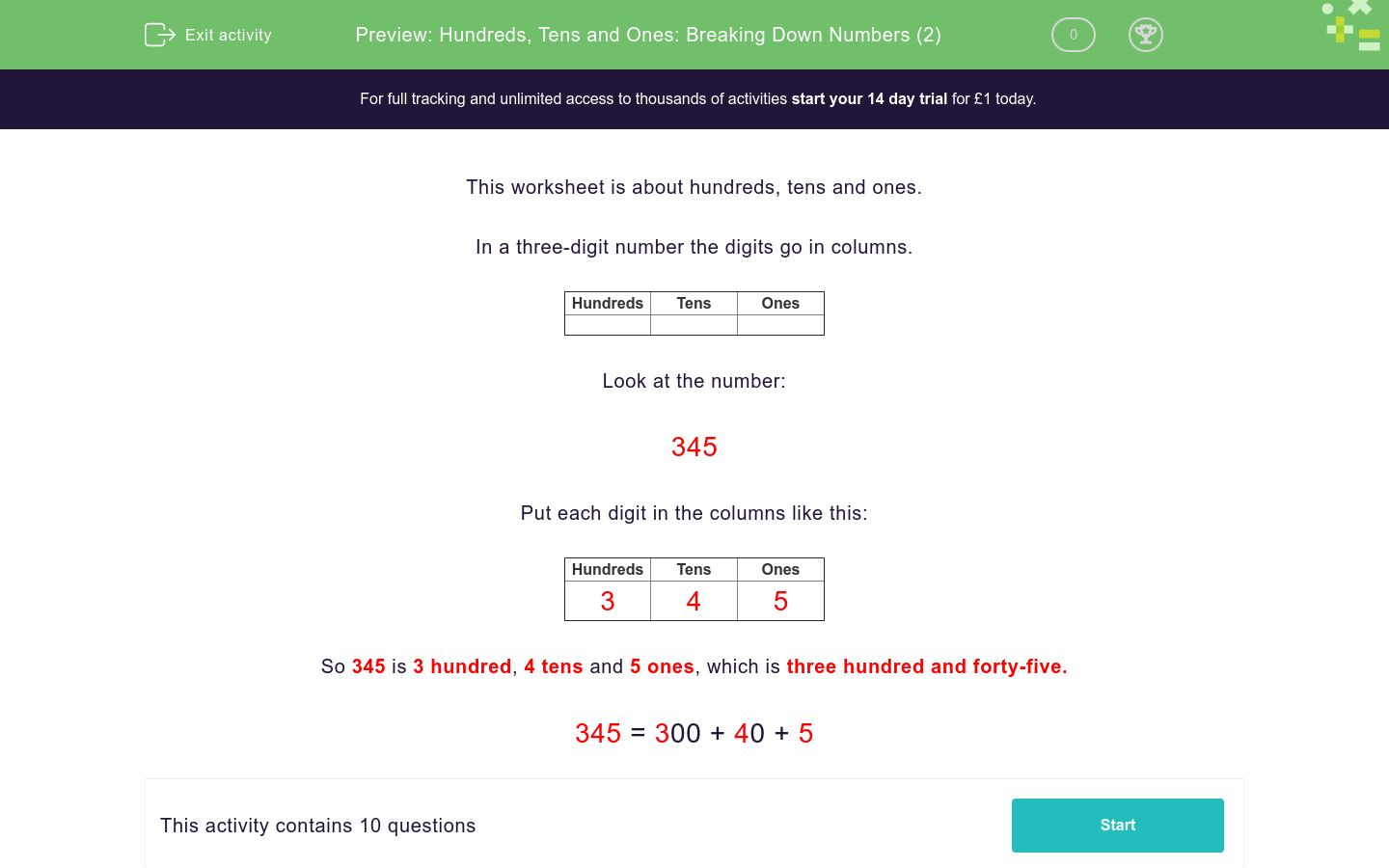# Hundreds, Tens and Ones: Breaking Down Numbers (2)

In this worksheet, students break a number down into its HTU values.Key stage:  KS 2

Curriculum topic:   Number: Number and Place Value

Curriculum subtopic:   Recognise Hundreds, Tens and Ones

Difficulty level:### QUESTION 1 of 10

This worksheet is about hundreds, tens and ones.

In a three-digit number the digits go in columns.

 Hundreds Tens Ones

Look at the number:

345

Put each digit in the columns like this:

 Hundreds Tens Ones 3 4 5

So 345 is 3 hundred, 4 tens and 5 ones, which is three hundred and forty-five.

345 = 300 + 40 + 5

690 = __00 + __0 + __

600 + 90 + 0

000 + 600 + 9

9000 + 60 + 0

974 = __00 + __0 + __

900 + 70 + 4

700 + 900 + 4

9000 + 70 + 4

893 = __00 + __0 + __

800 + 30 + 9

900 + 800 + 9

800 + 90 + 3

412 = __00 + __0 + __

400 + 10 + 2

20 + 400 + 1

4000 + 10 + 2

545 = __00 + __0 + __

5000 + 40 + 5

400 + 50 + 4

500 + 40 + 5

787 = __00 + __0 + __

700 + 80 + 7

800 + 700 + 7

7000 + 80 + 7

493 = __00 + __0 + __

4000 + 90 + 3

900 + 30 + 4

400 + 90 + 3

531 = __00 + __0 + __

500 + 30 + 1

300 + 500 + 1

5000 + 30 + 1

590 = __00 + __0 + __

500 + 90 + 9

900 + 500 + 0

500 + 90 + 0

953 = __00 + __0 + __

900 + 50 + 3

500 + 30 + 9

9000 + 50 + 3

• Question 1

690 = __00 + __0 + __

600 + 90 + 0
EDDIE SAYS

6 hundred, 9 tens and 0 ones

• Question 2

974 = __00 + __0 + __

900 + 70 + 4
EDDIE SAYS

9 hundred, 7 tens and 4 ones

• Question 3

893 = __00 + __0 + __

800 + 90 + 3
EDDIE SAYS

8 hundred, 9 tens and 3 ones

• Question 4

412 = __00 + __0 + __

400 + 10 + 2
EDDIE SAYS

4 hundred, 1 ten and 2 ones

• Question 5

545 = __00 + __0 + __

500 + 40 + 5
EDDIE SAYS

5 hundred, 4 tens and 5 ones

• Question 6

787 = __00 + __0 + __

700 + 80 + 7
EDDIE SAYS

7 hundred, 8 tens and 7 ones

• Question 7

493 = __00 + __0 + __

400 + 90 + 3
EDDIE SAYS

4 hundred, 9 tens and 3 ones

• Question 8

531 = __00 + __0 + __

500 + 30 + 1
EDDIE SAYS

5 hundred, 3 tens and 1 one

• Question 9

590 = __00 + __0 + __

500 + 90 + 0
EDDIE SAYS

5 hundred, 9 tens and 0 ones

• Question 10

953 = __00 + __0 + __

900 + 50 + 3
EDDIE SAYS

9 hundred, 5 tens and 3 ones

---- OR ----

Sign up for a £1 trial so you can track and measure your child's progress on this activity.

### What is EdPlace?

We're your National Curriculum aligned online education content provider helping each child succeed in English, maths and science from year 1 to GCSE. With an EdPlace account you’ll be able to track and measure progress, helping each child achieve their best. We build confidence and attainment by personalising each child’s learning at a level that suits them.

Get started